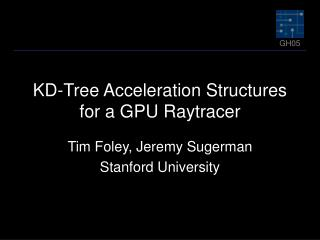# KD-Tree Acceleration Structures for a GPU Raytracer - PowerPoint PPT PresentationDownload PresentationKD-Tree Acceleration Structures for a GPU Raytracer

KD-Tree Acceleration Structures for a GPU Raytracer
Download Presentation## KD-Tree Acceleration Structures for a GPU Raytracer

- - - - - - - - - - - - - - - - - - - - - - - - - - - E N D - - - - - - - - - - - - - - - - - - - - - - - - - - -
##### Presentation Transcript

1. KD-Tree Acceleration Structures for a GPU Raytracer Tim Foley, Jeremy Sugerman Stanford University

2. Motivation • Accelerated raytracing • On commodity HW • Production rendering • Real-time applications? • Performance trend • 9800 XT : 170M ray-triangle intersects/s • X800 XT PE: 350M ray-triangle intersects/s

3. GPU Raytracing • Promising early results • Simple scenes • Uniform grid • Problems with complex scenes • Hierarchical accelerator (kd-tree) • Improve scalability

4. Outline • Background • GPU Raytracing • KD-Tree Algorithm • KD-Restart, KD-Backtrack • Results • Future Work

5. Background • RayEngine [Carr et al. 2002] • Parallel ray-triangle intersection • Host controls culling • [Purcell et al. 2002] • Entire raytracing pipeline • Many rays required for efficiency • Uniform Grid

6. Why not KD-Tree? • Uniform grid acceleration structure • Regular structure = efficient traversal • Regular structure = poor partitioning • KD-Trees • Adapt to scene complexity • Compact storage, efficient traversal • “Best” for CPU raytracing [Havran 2000]

7. X Y Z A C B D KD-Tree tmin Z X B Y D C A tmax

8. Z X B X Y Y Z D C A A C B D KD-Tree Traversal

9. Per-Fragment Stacks • Parallel (per-ray) push • No indexed write in fragment program • Per-ray stack storage • [Ernst et al. 2004] • Emulate push with extra passes • Impractical, slow

10. Our Contribution • Stackless kd-tree traversal algorithms • KD-Restart • KD-Backtrack

11. Z X B X Y Y Z D C A A C B D Observation Current leaf’s tmax Next leaf’s tmin =

12. Z X B Y D C A KD-Restart • Standard traversal • Omit stack operations • Proceed to 1st leaf • If no intersection • Advance (tmin,tmax) • Restart from root • Proceed to next leaf

13. KD-Restart • Restart traversal after each leaf • m leaves • Average depth d • Cost O(m*d) • Balanced tree of n nodes • Upper bound: O(n log(n)) • Standard algorithm: O(n) • Expected: O( log(n) )

14. Z X B X Y Y Z D C A A C B D Observation Ancestor of A isparent of Z

15. Z X B Y D C A KD-Backtrack • If no intersection • Advance (tmin, tmax) • Start backtracking • If node intersects (tmin, tmax) • Resume traversal • Proceed to next leaf

16. KD-Backtrack • Backtrack after leaf • Revisits previous nodes • At most twice: from left, right • Within constant factor of standard traversal • Upper bound: O(n) • Expected: O( log(n) ) • Requires additional storage • Parent pointers • Bounding boxes for internal nodes

17. Implementation • Built GPU raytracer in Brook [Buck et al.] • 4 intersection schemes: • Brute Force • Uniform Grid • KD-Restart • KD-Backtrack

18. Scenes Stanford Bunny 69451 triangles Cornell Box 32 triangles BART Robots 71708 triangles BART Kitchen 110561 triangles

19. Results Box Bunny Robots Kitchen 12.9 Relative speedup over brute-force intersection.

20. Results Rays in each state throughout traversal.

21. Discussion • Absolute performance • Trails best CPU implementations 5-6x • Sources of inefficiency • Load balancing • Data reuse

22. Load Balancing • Subset of rays intersecting, traversing • Occlusion queries to select kernel • Early-Z to cull inactive rays • Approximately 5x overhead • Query, kernel switch overhead • Worse with fewer rays

23. Data Reuse • Every kernel • Loads ray origin/direction • Load/Store traversal state • Consumes streaming bandwidth • We are bandwidth-limited • CPU implementation stores these in registers

24. Branching • Merge multiple passes into larger kernel • Fragment branches for load balancing • Avoid load/store of reused data • Current branching has high overhead • Shifts efficiency burden to HW

25. Conclusion • Stackless Traversal • Allows efficient GPU kd-tree • Scales to larger, more complex scenes • Future Work • Changes in HW • Alternative acceleration structures • “Out-of-core” scenes • Dynamic scenes

26. Acknowledgements • Tim Purcell (NVIDA) • Streaming raytracer • Mark Segal (ATI) • Demo machine • NVIDIA, ATI : HW • DARPA, Rambus : Funding

27. Questions